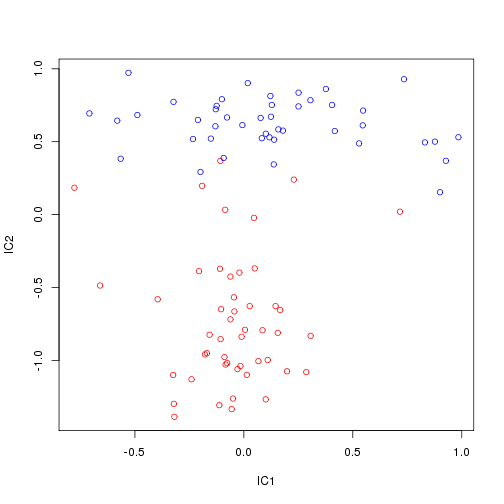library(DESeq); library(statmod); library(pcaMethods); library(fastICA)


## Identifying highly variable genes

We'll start with the count matrix that was prepared in the previous exercise.

load("counts.RData")


In case something went wrong with the previous exercise, you can load a clean version by issuing the following command: load(“/groups/pklab/scw2014/tutorials/counts.RData”)

To get normalized expression magnitudes, estimate library size using DESeq and convert counts to expression magnitudes:

(note: $$counts$$ variable was defined in differential_expression.Rmd)

require(DESeq)
lib.size <- estimateSizeFactorsForMatrix(counts)
ed <- t(t(counts)/lib.size)


Calculate estimates of variance, coefficient of variation

means <- rowMeans(ed)
vars <- apply(ed,1,var)
cv2 <- vars/means^2
par(mar=c(3.5,3.5,1,1),mgp=c(2,0.65,0),cex=0.9)
smoothScatter(log(means),log(cv2))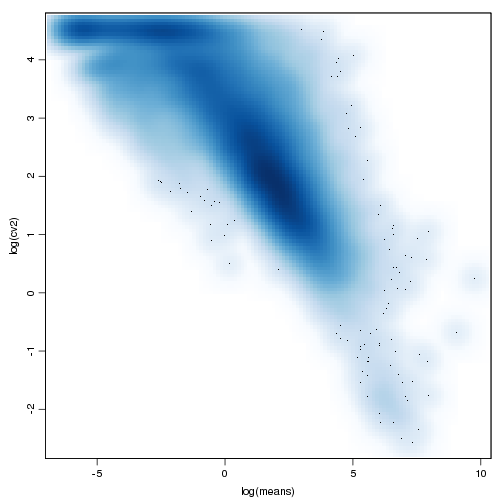Now fit a regression line based on the controls:

require(statmod)
minMeanForFit <- unname( quantile( means[ which( cv2 > .3 ) ], .95 ) )
useForFit <- means >= minMeanForFit # & spikeins
fit <- glmgam.fit( cbind( a0 = 1, a1tilde = 1/means[useForFit] ),cv2[useForFit] )
a0 <- unname( fit$coefficients["a0"] ) a1 <- unname( fit$coefficients["a1tilde"])
fit$coefficients  ## a0 a1tilde ## 0.7307 263.0521  Now add the fit and the 95% confidence interval to our plot: # repeat previous plot par(mar=c(3.5,3.5,1,1),mgp=c(2,0.65,0),cex=0.9); smoothScatter(log(means),log(cv2)); xg <- exp(seq( min(log(means[means>0])), max(log(means)), length.out=1000 )) vfit <- a1/xg + a0 # add fit line lines( log(xg), log(vfit), col="black", lwd=3 ) df <- ncol(ed) - 1 # add confidence interval lines(log(xg),log(vfit * qchisq(0.975,df)/df),lty=2,col="black") lines(log(xg),log(vfit * qchisq(0.025,df)/df),lty=2,col="black")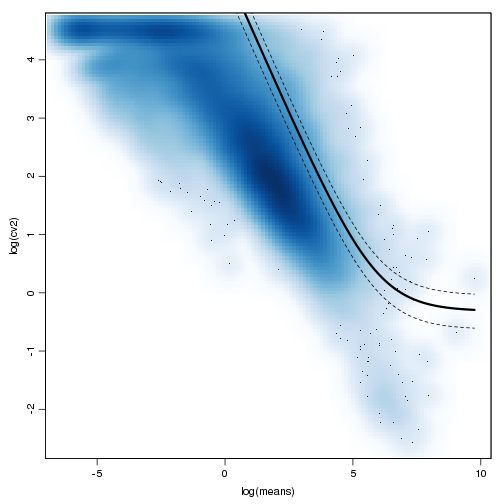Rank genes by the significance of deviation from the fit afit <- a1/means+a0 varFitRatio <- vars/(afit*means^2) varorder <- order(varFitRatio,decreasing=T) oed <- ed[varorder,] # save for the next exercise save(oed,file="oed.RData") # repeat previous plot par(mar=c(3.5,3.5,1,1),mgp=c(2,0.65,0),cex=0.9); smoothScatter(log(means),log(cv2)); lines( log(xg), log(vfit), col="black", lwd=3 ); lines(log(xg),log(vfit * qchisq(0.975,df)/df),lty=2,col="black"); lines(log(xg),log(vfit * qchisq(0.025,df)/df),lty=2,col="black"); # add top 100 genes points(log(means[varorder[1:100]]),log(cv2[varorder[1:100]]),col=2)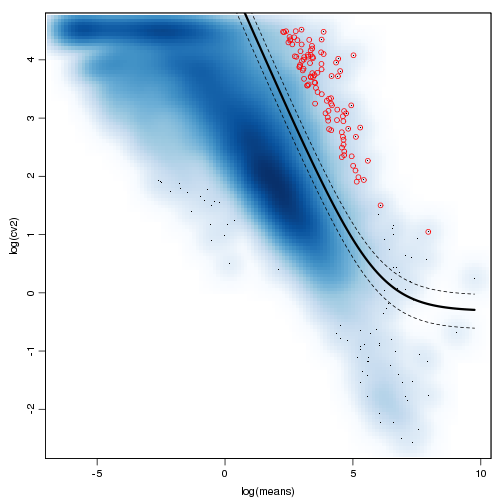We can also evaluate statistical significance of the deviation pval <- pchisq(varFitRatio*df,df=df,lower.tail=F) adj.pval <- p.adjust(pval,"fdr") sigVariedGenes <- adj.pval<1e-3; table(sigVariedGenes)  ## sigVariedGenes ## FALSE TRUE ## 17115 257  Look at how the most variable genes are expressed … m <- oed[1:50,] heatmap(m/apply(m,1,max),zlim=c(0,1),col=gray.colors(100),Rowv=NA,Colv=NA,labRow=NA,scale="none",ColSideColors=ifelse(grepl("ES",colnames(m)),"red","blue"))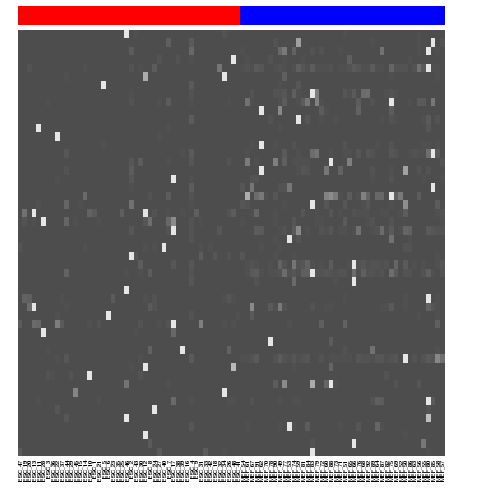Let's apply Winsorization procedure to the expression matrix winsorize <- function (x, fraction=0.05) { if(length(fraction) != 1 || fraction < 0 || fraction > 0.5) { stop("bad value for 'fraction'") } lim <- quantile(x, probs=c(fraction, 1-fraction)) x[ x < lim ] <- lim x[ x > lim ] <- lim x } # winsorize to remove 2 most extreme cells (from each side) wed <- t(apply(ed, 1, winsorize, fraction=2/ncol(ed))) # now let's recalculate the most variable genes with the winsorized matrix (wed) means = rowMeans(wed); vars = apply(wed,1,var); cv2 <- vars/means^2 useForFit <- means >= unname( quantile( means[ which( cv2 > .3 ) ], .95 ) ) fit <- glmgam.fit( cbind( a0 = 1, a1tilde = 1/means[useForFit] ),cv2[useForFit] ) afit <- fit$coef["a1tilde"]/means+fit$coef["a0"] vfit <- fit$coef["a1tilde"]/xg+fit$coef["a0"] varFitRatio <- vars/(afit*means^2) varorder <- order(varFitRatio,decreasing=T) oed <- wed[varorder,] # save for the next exercise save(oed,file="oed_win.RData") xg <- exp(seq( min(log(means[means>0])), max(log(means)), length.out=1000 )) par(mar=c(3.5,3.5,1,1),mgp=c(2,0.65,0),cex=0.9); smoothScatter(log(means),log(cv2)); lines( log(xg), log(vfit), col="black", lwd=3 ); lines(log(xg),log(vfit * qchisq(0.975,df)/df),lty=2,col="black"); lines(log(xg),log(vfit * qchisq(0.025,df)/df),lty=2,col="black"); # add top 100 genes points(log(means[varorder[1:100]]),log(cv2[varorder[1:100]]),col=2)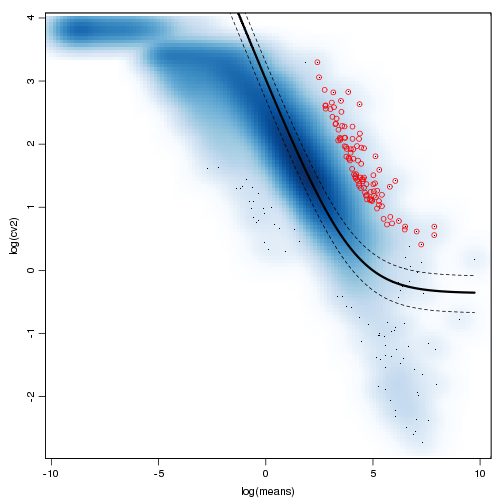Let's redraw the top 50 most variable genes: m <- oed[1:50,] heatmap(m/apply(m,1,max),zlim=c(0,1),col=gray.colors(100),Rowv=NA,Colv=NA,labRow=NA,scale="none",ColSideColors=ifelse(grepl("ES",colnames(m)),"red","blue"))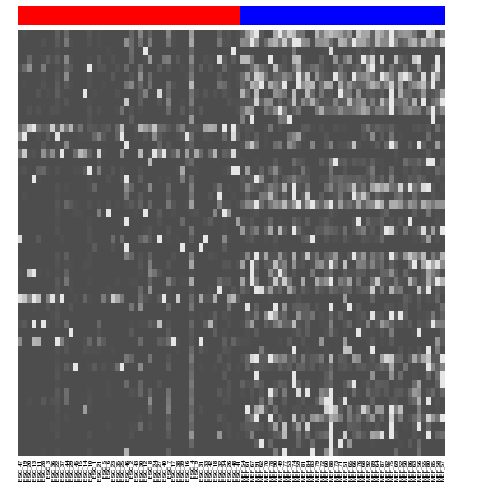## Heterogeneity analysis through clustering Cluster cells based on correlation of expression values .. plot(hclust(as.dist(1-cor(ed))))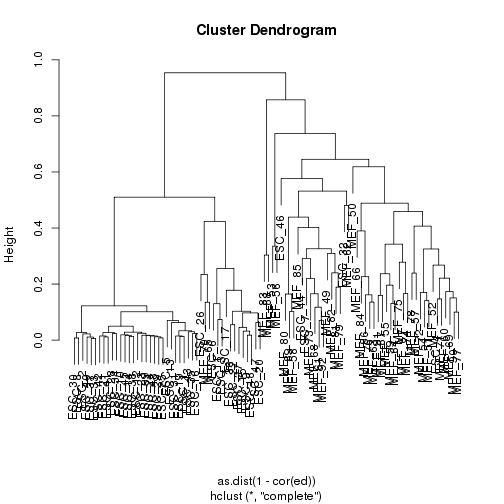Let's color nodes according to cell type. # labeling function colLab <- function(n) { if(is.leaf(n)) { a <- attributes(n); attr(n,"nodePar") <- c(a$nodePar,list(lab.col=ifelse(grepl("ES",a$label),"red","blue"))); } n } clust <- hclust(as.dist(1-cor(ed)),method="ward");  ## The "ward" method has been renamed to "ward.D"; note new "ward.D2"  plot(dendrapply(as.dendrogram(clust),colLab),cex=0.7)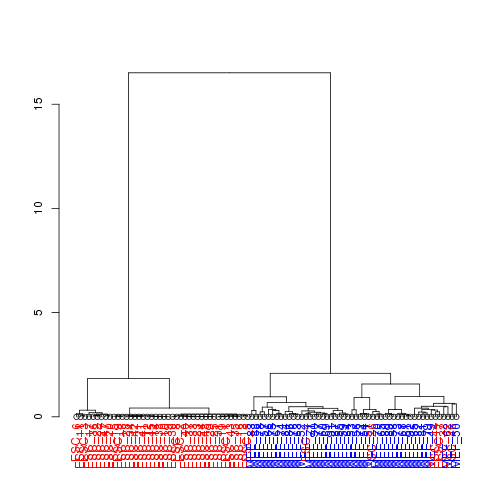Use top 100 most variable genes from the winsorized matrix: clust <- hclust(as.dist(1-cor(oed[1:100,],method="p")),method="ward");  ## The "ward" method has been renamed to "ward.D"; note new "ward.D2"  plot(dendrapply(as.dendrogram(clust),colLab),cex=0.7)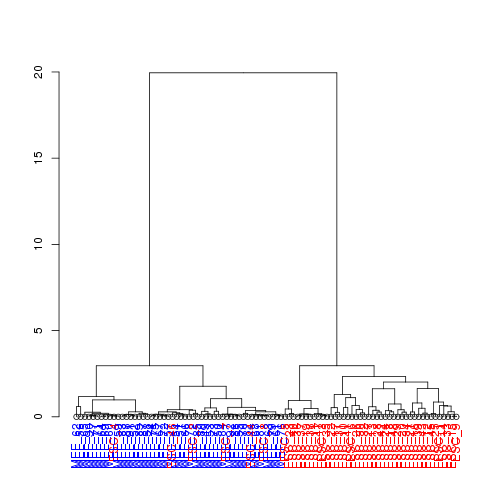We can also use weighted distance measures to reduce the contribution of the technical noise. Please see SCDE tutorial for further details. Note: the block below fits error models for all 90+ cells, so it takes a while. require(scde) scde.fitted.model <- scde.error.models(counts=counts,n.cores=n.cores,save.model.plots=F) scde.prior <- scde.expression.prior(models=scde.fitted.model,counts=counts) jp <- scde.posteriors(models=scde.fitted.model,counts,scde.prior,return.individual.posterior.modes=T,n.cores=n.cores) jp$jp.modes <- log(as.numeric(colnames(jp$jp)))[max.col(jp$jp)]
p.mode.fail <- scde.failure.probability(models=scde.fitted.model,magnitudes=jp$jp.modes) p.self.fail <- scde.failure.probability(models=scde.fitted.model,counts=counts) # weight matrix matw <- 1-sqrt(p.self.fail*sqrt(p.self.fail*p.mode.fail)) # magnitude matrix (using individual posterior modes here) mat <- log10(exp(jp$modes)+1);
# weighted distance
cell.names <- colnames(counts); names(cell.names) <- cell.names;

require(boot)
mode.fail.dist <- as.dist(1-do.call(rbind,mclapply(cell.names,function(nam1) {
unlist(lapply(cell.names,function(nam2) {
corr(cbind(mat[,nam1],mat[,nam2]),w=sqrt(sqrt(matw[,nam1]*matw[,nam2])))
}))
},mc.cores=n.cores)),upper=F);

save(mode.fail.dist,file="mode.fail.dist.RData")


The calculation above takes some time, so let's just use a pre-calculated distance:

load("/groups/pklab/scw2014/tutorials/mode.fail.dist.RData")
clust <- hclust(mode.fail.dist,method="ward");

## The "ward" method has been renamed to "ward.D"; note new "ward.D2"

plot(dendrapply(as.dendrogram(clust),colLab),cex=0.7)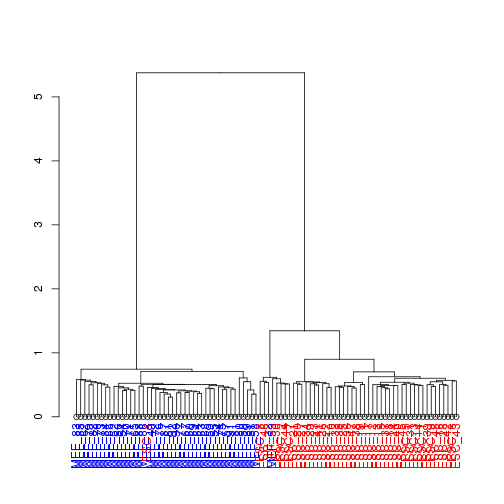## Using PCA and ICA to separate subpopulations

First, let's try applying PCA to the complete set of genes

require(pcaMethods)
pcs <- pca(oed,nPcs=5)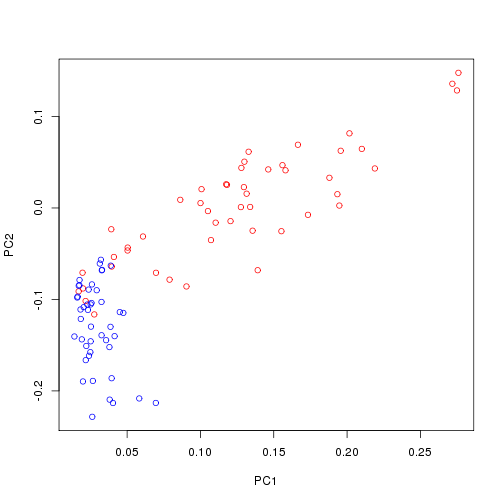We can get similarly good separation with just the top 100 most variable genes

pcs <- pca(oed[1:100,],nPcs=5)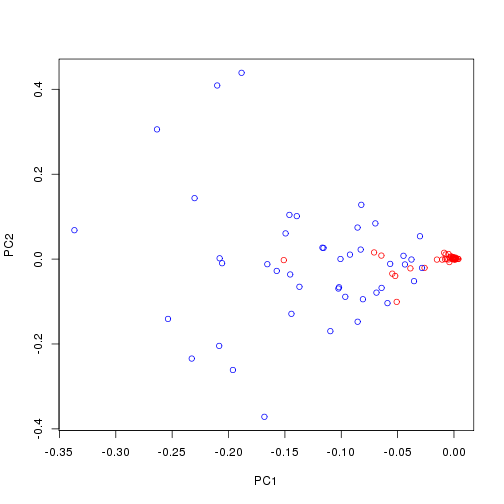ICA is another popular dimensionality reduction approach:

require(fastICA)
ics <- fastICA(oed[1:100,],n.comp=4)
plot(ics$A[1,],ics$A[2,],col=ifelse(grepl("ES",colnames(oed)),"red","blue"),xlab="IC1",ylab="IC2")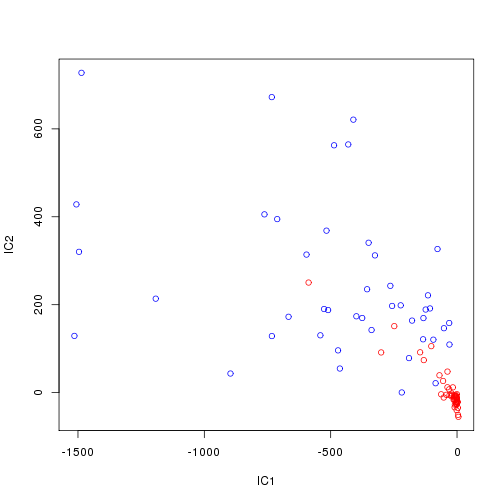plot(ics$A[3,],ics$A[4,],col=ifelse(grepl("ES",colnames(oed)),"red","blue"),xlab="IC1",ylab="IC2")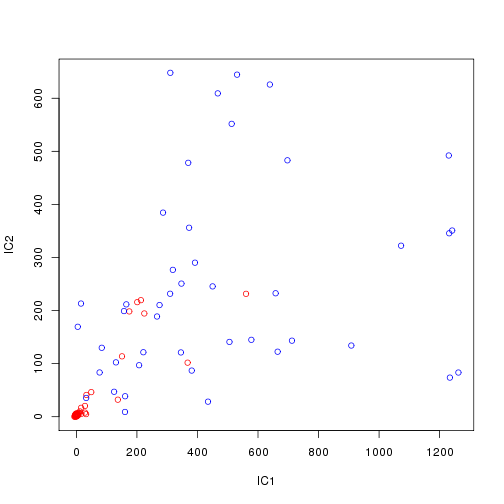ics <- fastICA(log(oed[1:100,]+1),n.comp=4)
plot(ics$A[1,],ics$A[2,],col=ifelse(grepl("ES",colnames(oed)),"red","blue"),xlab="IC1",ylab="IC2")